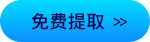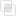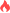Python SymPy求极值

Python2021-03-30 15:37:181085浏览 · 0收藏 · 0评论SymPy是Python符号计算库。其目标是成为一个功能齐全的计算机代数系统，代码保持简洁，易于理解和扩展。Python是完全由Python编写的，不依赖外部库。

1、求极限、求导、求偏导以及带值求导

```import sympy
#求极限
#设置符号变量Symbol只能创建一个变量 symbols 可一次定义多个变量
x1,x2,x3,x4=sympy.symbols('x1,x2,x3,x4')
#创建函数建立方程式
def F(t):
return sympy.sin(t)/t
def N(t):
return (x1**3+3*x1**2+1)/(4*x1**3+2*+3)
#调用limit求极限
limF=sympy.limit(F(x1),x1,0)
limN=sympy.limit(N(x1),x1,sympy.oo)
print("x1趋于0的极限为{}".format(limF))
print("x1趋于0的极限为{}".format(limN))
#求导
#创建求导函数
def S(t):
return sympy.sec(t) #正割
def S1(x):
return 2*x**4+2
#调用diff函数求导
s=sympy.diff(S(x1),x1).subs(x1,1) #subs 带值求导
print('S在1处的导数为{}'.format(s))
#求多阶导数 2阶
s1=sympy.diff(S1(x1),x1,2)
#带值计算
print("S1的二阶导数{}   带入值2计算为{}".format(s1,s1.subs(x1,2)))
#建立求偏导函数
def PD(x,y,z):
return sympy.sin(x+pow(y,2)-sympy.exp(z))
#对x求偏导
x=sympy.diff(PD(x1,x2,x3),x1)
# print(x.subs(x1,2))
#对y求偏导
y=sympy.diff(PD(x1,x2,x3),x2)
#对z求偏导
z=sympy.diff(PD(x1,x2,x3),x3,2)
print("x的偏导为{}\ny的偏导为{}\nz的二次偏导为{}".format(x,y,z))

2、建立极限表达式

```from sympy import Limit, sin, Symbol
from sympy.abc import x
Limit(sin(x)/x, x, 0) # 这是一个极限表达式，不执行计算
Limit(1/x, x, 0, dir='-') # 这也是一个极限表达式，不执行计算```python切片步长怎样实现python字符串格式化的方法整理python语句和缩进的实现python变量如何拼接python常量是什么python random中的随机函数python函数式编程如何理解python loguru如何记录日志python使用loguru操作日志python input()的特性python while循环三要素python列表中有哪些内置函数python系统内置方法如何获取python列表追加元素出错的解决python匿名函数lambda的注意点python列表的数据类型分析python分数如何使用877

python tempfile创建文件378

python finally语句如何使用205